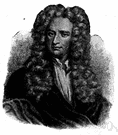# newton

(redirected from Attonewton)
Also found in: Thesaurus, Medical, Encyclopedia.
Related to Attonewton: Yoctonewton, Zeptonewton, Femtonewton, Piconewton

## new·ton

(no͞ot′n, nyo͞ot′n)
n. Abbr. N
The SI-derived unit of force required to accelerate a mass of one kilogram one meter per second per second, equal to 100,000 dynes. See Table at measurement.

[After Sir Isaac Newton.]

## newton

(ˈnjuːtən)
n
(Units) the derived SI unit of force that imparts an acceleration of 1 metre per second to a mass of 1 kilogram; equivalent to 105 dynes or 7.233 poundals. Symbol: N
[C20: named after Sir Isaac Newton]

## Newton

(ˈnjuːtən)
n
(Astronomy) one of the deepest craters on the moon, over 7300 m deep and about 112 km in diameter, situated in the SE quadrant

## Newton

(ˈnjuːtən)
n
(Biography) Sir Isaac. 1642–1727, English mathematician, physicist, astronomer, and philosopher, noted particularly for his law of gravitation, his three laws of motion, his theory that light is composed of corpuscles, and his development of calculus independently of Leibnitz. His works include Principia Mathematica (1687) and Opticks (1704)

## new•ton

(ˈnut n, ˈnyut n)

n.
the SI unit of force, equal to the force that produces an acceleration of one meter per second per second on a mass of one kilogram.
[1900–05; after I. Newton]

## New•ton

(ˈnut n, ˈnyut n)

n.
1. Sir Isaac, 1642–1727, English physicist and mathematician.
2. a city in E Massachusetts, near Boston. 82,230.

## new·ton

(no͞ot′n)
A unit used to measure force. One newton is equal to the force needed to accelerate a mass of one kilogram one meter per second per second.

## newton

1. The unit of force giving a mass of 1 kg an acceleration of about 1 m per second per second.
2. (N) A unit of force which, when applied, accelerates a mass of one kilogram by one meter per second per second. This unit has replaced the dyne: 1 N = 105 dynes. Named after Isaac Newton (1642–1727).
ThesaurusAntonymsRelated WordsSynonymsLegend:
 Noun 1Newton - English mathematician and physicist; remembered for developing the calculus and for his law of gravitation and his three laws of motion (1642-1727) 2 newton - a unit of force equal to the force that imparts an acceleration of 1 m/sec/sec to a mass of 1 kilogram; equal to 100,000 dynesNforce unit - a unit of measurement of physical forcedyne - a unit of force equal to the force that imparts an acceleration of 1 cm/sec/sec to a mass of 1 gramsthene - a unit of force equal to 1000 newtons
Translations
njutn
ニュートン
niuton
newton

## newton

[ˈnjuːtn] Nnewton m, neutonio m
Site: Follow: Share:
Open / Close